# Samacheer Kalvi Books: Tamilnadu State Board Text Books Solutions

## Samacheer Kalvi 12th Physics Chapter 3 Magnetism and Magnetic Effects of Electric Current Notes PDF Download: Tamil Nadu STD 12th Physics Chapter 3 Magnetism and Magnetic Effects of Electric Current NotesSamacheer Kalvi 12th Physics Chapter 3 Magnetism and Magnetic Effects of Electric Current Notes PDF Download: Tamil Nadu STD 12th Physics Chapter 3 Magnetism and Magnetic Effects of Electric Current Notes

## Samacheer Kalvi 12th Physics Chapter 3 Magnetism and Magnetic Effects of Electric Current Notes PDF Download

We bring to you specially curated Samacheer Kalvi 12th Physics Chapter 3 Magnetism and Magnetic Effects of Electric Current Notes PDF which have been prepared by our subject experts after carefully following the trend of the exam in the last few years. The notes will not only serve for revision purposes, but also will have several cuts and easy methods to go about a difficult problem.

 Board Tamilnadu Board Study Material Notes Class Samacheer Kalvi 12th Physics Subject 12th Physics Chapter Chapter 3 Magnetism and Magnetic Effects of Electric Current Format PDF Provider Samacheer Kalvi Books

## How to Download Samacheer Kalvi 12th Physics Chapter 3 Magnetism and Magnetic Effects of Electric Current Notes PDFs?

2. Click on the Samacheer Kalvi 12th Physics Notes PDF.
3. Look for your preferred subject.
4. Now download the Samacheer Kalvi 12th Physics Chapter 3 Magnetism and Magnetic Effects of Electric Current notes PDF.

## Download Samacheer Kalvi 12th Physics Chapter 3 Magnetism and Magnetic Effects of Electric Current Chapterwise Notes PDF

Samacheer Kalvi 12th Physics Magnetism and Magnetic Effects of Electric Current Multiple Choice Questions

12th Physics Chapter 3 Book Back
The magnetic field at the center O of the following current loop is-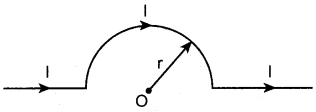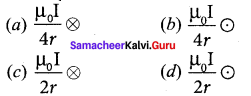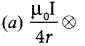12th Physics 3rd Lesson Book Back
An electron moves straight inside a charged parallel plate capacitor of uniform charge density σ The time taken by the electron to cross the parallel plate capacitor when the plates of the capacitor are kept under constant magnetic field of induction B⃗  is-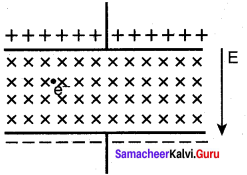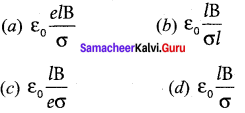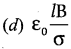12th Physics Lesson 3 Book Back
The force experienced by a particle having mass m and charge q accelerated through a potential difference V when it is kept under perpendicular magnetic field B⃗  is-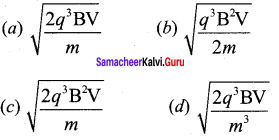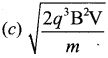Samacheer Kalvi Guru 12th Physics Question 4. A circular coil of radius 5 cm and 50 turns carries a current of 3 ampere. The magnetic dipole moment of the coil is- (a) 1.0 amp – m2 (b) 1.2 amp – m2 (c) 0.5 amp – m2 (d) 0.8 amp – m2
Answer: (b) 1.2 amp – m2

Magnetic Effect Of Electric Current Class 12 Numericals Pdf Question 5. A thin insulated wire forms a plane spiral of N = 100 tight turns carrying a current 1 = 8 m A (milli ampere). The radii of inside and outside turns are a = 50 mm and b = 100 mm respectively. The magnetic induction at the center of the spiral is (a) 5 μT (b) 7 μT (c) 8 μT (d) 10 μT

Samacheerkalvi.Guru 12th Physics Question 6. Three wires of equal lengths are bent in the form of loops. One of the loops is circle, another is a semi-circle and the third one is a square. They are placed in a uniform magnetic field and same electric current is passed through them. Which of the following loop configuration will experience greater torque ? (a) circle (b) semi-circle (c) square (d) all of them

Samacheer Kalvi.Guru 12th Physics Question 7. Two identical coils, each with N turns and radius R are placed coaxially at a distance R as shown in the figure. If I is the current passing through the loops in the same direction, then the magnetic field at a point P which is at exactly at R2 distance between two coils is-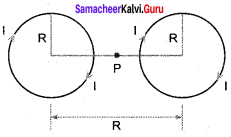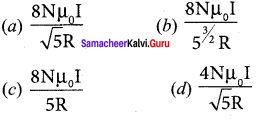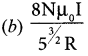Samacheer Kalvi Guru Physics Question 8. A wire of length l carries a current I along the Y direction and magnetic field is given by B⃗  = β√3 (i^+j^+k^). The magnitude of Lorentz force acting on the wire is-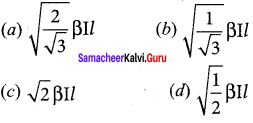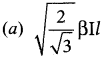Physics Solution Class 12 Samacheer Kalvi Question 9. A bar magnet of length l and magnetic moment M is bent in the form of an arc as shown in figure. The new magnetic dipole moment will be- (NEET 2014)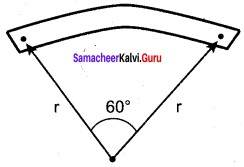(a) M (b) π3 M (c) 2π M (d) 12 M

Samacheer Kalvi 12th Physics Question 10. A non-conducting charged ring of charge q, mass m and radius r is rotated with constant angular speed ω. Find the ratio of its magnetic moment with angular momentum is (a) qm M (b) 2q3 M (c) q2m M (d) q4m M

Question 11. The BH curve for a ferromagnetic material is shown in the figure. The material is placed inside a long solenoid which contains 1000 turns/ cm. The current that should be passed in the solenoid to demagnetize the ferromagnet completely is-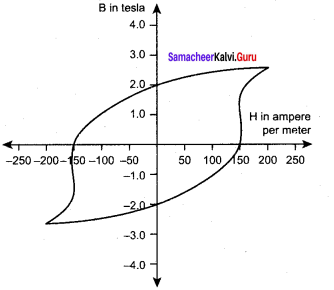(a) 1.00 m A(milli ampere) (b) 1.25 mA (c) 1.50 mA (d) 1.75 mA

Question 12. Two short bar magnets have magnetic moments 1.20 Am2 and 1.00 Am2respectively. They are kept on a horizontal table parallel to each other with their north poles pointing towards the south. They have a common magnetic equator and are separated by a distance of 20.0 cm. The value of the resultant horizontal magnetic induction at the mid-point O of the line joining their centers is (Horizontal components of Earth’s magnetic induction is 3.6 x 10-5 Wb m-2) (NSEP 2000-2001) (a) 3.60 x 10-5 Wb m-1 (b) 3.5 x 10-5 Wb m-1 (c) 2.56 x 10-4 Wb m-1 (d) 2.2 x 10-4 Wb m-1
Answer: (c) 2.56 x 10-4 Wb m-1

Question 13. The vertical component of Earth’s magnetic field at a place is equal to the horizontal component. What is the value of angle of dip at this place? (a) 30° (b) 45° (c) 60° (d) 90°

Question 14. A flat dielectric disc of radius R carries an excess charge on its surface. The surface charge density is σ. The disc rotates about an a × is perpendicular to its plane passing through the center with angular velocity ω. Find the magnitude of the torque on the disc if it is placed in a uniform magnetic field whose strength is B which is directed perpendicular to the a × is of rotation (a) 14 σωπ BR (b) 14 σωπ BR2 (c) 14 σωπ BR3 (d) 14 σωπ BR4

Question 15. A simple pendulum with charged bob is oscillating with time period T and let θ be the angular displacement. If the uniform magnetic field is switched ON in a direction perpendicular to the plane of oscillation then- (a) time period will decrease but θ will remain constant (b) time period remain constant but θ will decrease (c) both T and θ will remain the same (d) both T and θ will decrease
Answer: (c) both T and θ will remain the same

Magnetism and Magnetic Effects of Electric Current Short

Question 1. What is meant by magnetic induction?
Answer: The magnetic induction (total magnetic field) inside the specimen B⃗  is equal to the sum of the magnetic field B⃗ 0 produced in vacuum due to the magnetising field and the magnetic field B⃗ m due to the induced magnetisation of the substance. B⃗  = B⃗ 0 + B⃗ m = µ0H⃗  + µ0I⃗  = µ0 (H⃗  + I⃗ ) = (H⃗  + I⃗ )

Question 2. Define magnetic flux.
Answer: The number of magnetic field lines crossing per unit area is called magnetic flux B. ΦB = B⃗  .A⃗  = BA cos θ = B ⊥ A

Question 3. Define magnetic dipole moment.
Answer: The magnetic dipole moment is defined as the product of its pole strength and magnetic length. P⃗  = qm d⃗

Question 4. State Coulomb’s inverse law.
Answer: The force of attraction or repulsion between two magnetic poles is directly proportional to the product of their pole strengths and inversely proportional to the square of the distance between them. F→∝qmAqmbr2r^

Question 5. What is magnetic susceptibility?
Answer: It is defined as the ratio of the intensity of magnetisation (M⃗ ) induced in the material due to the magnetising field (H⃗ ) χm=∣∣∣M→H→∣∣∣

Question 6. State Biot-Savart’s law.
Answer: The magnitude of magnetic field dB⃗  at a point P at a distance r from the small elemental length taken on a conductor carrying current varies- (i) directly as the strength of the current I (ii) directly as the magnitude of the length element dl→ (iii) directly as the sine of the angle (say,θ) between dl→ and r^ . (iv) inversely as the square of the distance between the point P and length element dl→ This is expressed as dB∝Idlr2sinθ

Question 7. What is magnetic permeability?
Answer: Magnetic permeability: The magnetic permeability can be defined as the measure of ability of the material to allow the passage of magnetic field lines through it or measure of the capacity of the substance to take magnetisation or the degree of penetration of magnetic field through the substance.

Question 8. State Ampere’s circuital law.
Answer: The line integral of magnetic field over a closed loop is µ0 times net current enclosed by the loop.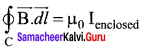Question 9. Compare dia, para and ferromagnetism.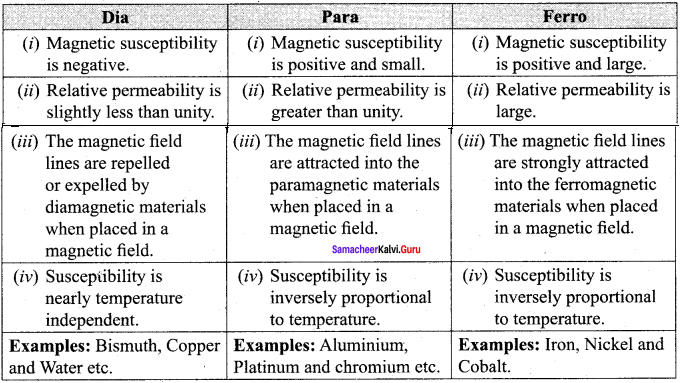Question 10. What is meant by hysteresis?
Answer: The phenomenon of lagging of magnetic induction behind the magnetising field is called hysteresis. Hysteresis means Tagging behind’.

Magnetism and Magnetic Effects of Electric Current Long

Question 1. Discuss Earth’s magnetic field in detail.
Answer: Gover suggested that the Earth’s magnetic field is due to hot rays coming out from the Sun. These rays will heat up the air near equatorial region. Once air becomes hotter, it rises above and will move towards northern and southern hemispheres and get electrified. This may be responsible to magnetize the ferromagnetic materials near the Earth’s surface.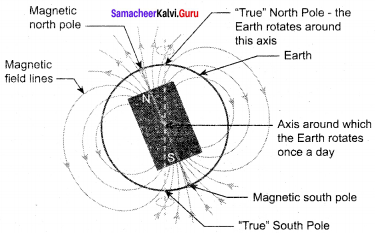The north pole of magnetic compass needle is attracted towards the magnetic south pole of the Earth which is near the geographic north pole. Similarly, the south pole of magnetic compass needle is attracted towards the geographic north pole of the Earth which is near magnetic north pole. There are three quantities required to specify the magnetic field of the Earth on its surface, which are often called as the elements of the Earth’s magnetic field. They are:

(a) Magnetic declination (D): The angle between magnetic meridian at a point and geographical meridian is called the declination or magnetic declination (D).

(b) Magnetic dip or inclination (I): The angle subtended by the Earth’s total magnetic field B⃗  with the horizontal direction in the magnetic meridian is called dip or magnetic inclination (I) at that point.

(c) The horizontal component of the Earth’s magnetic field (BH): The component of Earth’s magnetic field along the horizontal direction in the magnetic meridian is called horizontal component of Earth’s magnetic field, denoted by BH. Let BE be the net Earth’s magnetic field at a point P on the surface of the Earth. BE can be resolved into two perpendicular components. Horizontal component BH = BE cos I …… (1) Vertical component BV = BE sin I …… (2) Dividing the equation, we get tan I = BVBH …….(3)

(i) At magnetic equator The Earth’s magnetic field is parallel to the surface of the Earth (i.e., horizontal) which implies that the needle of magnetic compass rests horizontally at an angle of dip, I = 0°. B BE = BE Bv = 0 This implies that the horizontal component is maximum at equator and vertical component is zero at equator.

(ii) At magnetic poles. The Earth’s magnetic field is perpendicular to the surface of the Earth (i.e., vertical) which implies that the needle of magnetic compass rests vertically at an angle of dip, I = 90° Hence, BH = 0 Bv = BE This implies that the vertical component is maximum at poles and horizontal component is zero at poles.

Question 2. Deduce the relation for the magnetic induction at a point due to an infinitely long straight conductor carrying current.
Answer: Magnetic field due to long straight conductor carrying current: Consider a long straight wire NM with current I flowing from N to M. Let P be the point at a distance a from point O. Consider an element of length dl of the wire at a distance l from point O and r⃗  be the vector joining the element dl with the point P. Let θ be the angle between dl→ and r⃗ . Then, the magnetic field at P due to the element is dB⃗  = μ0Idl→4πr2 sinθ (unit vector perpendicular to dl→ and r⃗ ) …… (1) The direction of the field is perpendicular to the plane of the paper and going into it. This can be determined by taking the cross product between two vectors dl→ and r⃗  (let it be n^ ). The net magnetic field can be determined by integrating equation with proper limits.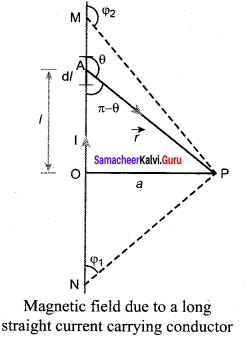B⃗  = ∫dB⃗  From the figure, in a right angle triangle PAO, tan (π – θ) = al l = atanθ (since tan (π – θ) = -tan θ) ⇒ 1tanθ l = a cot θ and r = a cosec θ differentiating, dl = a cosec2 θdθ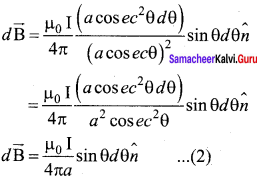This is the magnetic field at a point P due to the current in small elemental length. Note that we have expressed the magnetic field OP in terms of angular coordinate i.e. θ. Therefore, the net magnetic field at the point P which can be obtained by integrating dB⃗  by varying the angle from θ = (φ1 to θ = φ1 is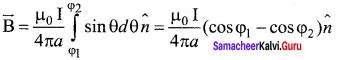For a an infinitely long straight wire, 1 = 0 and 2 = , the magnetic field is B⃗  = µ02πa n^ …… (3) Note that here n^ represents the unit vector from the point O to P.

Question 3. Obtain a relation for the magnetic induction at a point along the axis of a circular coil carrying current.
Answer: Magnetic field produced along the axis of the current carrying circular coil: Consider a current carrying circular loop of radius R and let I be the current flowing through the wire in the direction. The magnetic field at a point P on the axis of the circular coil at a distance z from its center of the coil O. It is computed by taking two diametrically opposite line elements of the coil each of length dl→ at C and D. Let r⃗  be the vector joining the current element (1 dl→) at C to the point P. PC = PD = T = R2+Z2−−−−−−−√ and angle ∠CPO = ∠DPO = θ According to Biot-Savart’s law, the magnetic field at P due to the current element I dl→ is dB⃗  = µ04π Idl⃗ ×r^r2 ….. (1) The magnitude of magnetic field due to current element l dl at C and D are equal because of equal distance from the coil. The magnetic field dB due to each current element I dl→ is resolved into two components; dB sin θ along y-direction and dB cos θ along z-direction. Horizontal components of each current element cancels out while the vertical components (dB cos θ k^) alone contribute to total magnetic field at the point P.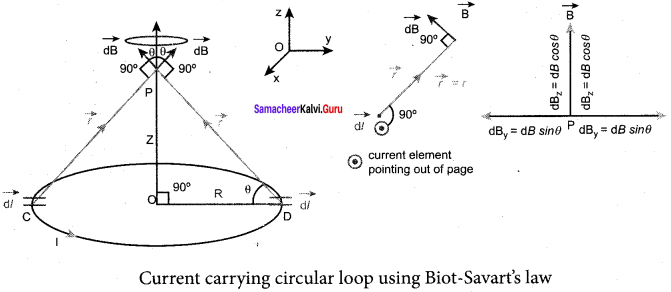If we integrate dl→ around the loop, dB⃗  sweeps out a cone, then the net magnetic field B⃗  at point P is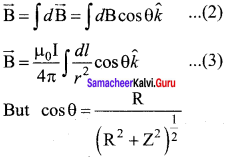Using Pythagorous theorem r2 = R2 + Z2 and integrating line element from 0 to 2πR, we get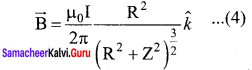Note that the magnetic field B⃗  points along the direction from the point O to P. Suppose if the current flows in clockwise direction, then magnetic field points in the direction from the point P to O.

Question 4. Compute the torque experienced by a magnetic needle in a uniform magnetic field.
Answer: Torque Acting on a Bar Magnet in Uniform Magnetic Field: Consider a magnet of length 21 of pole strength qm kept in a uniform magnetic field B⃗  Each pole experiences a force of magnitude qmB but acts in opposite direction.

Therefore, the net force exerted on the magnet is zero, so that there is no translatory motion. These two forces constitute a couple (about midpoint of bar magnet) which will rotate and try to align in the direction of the magnetic field B⃗ .

The force experienced by north pole, F⃗ N = qm B⃗  ……. (1) The force experienced by south pole, F⃗ S = qm B⃗  …….. (2) Adding equations (1) and (2), we get the net force acting on the dipole as F⃗  = F⃗ N + F⃗ S = O⃗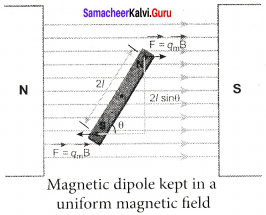This implies, that the net force acting on the dipole is zero, but forms a couple which tends to rotate the bar magnet clockwise (here) in order to align it along B⃗ . The moment of force or torque experienced by north and south pole about point O is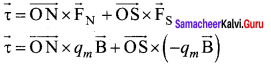By using right hand cork screw rule, we conclude that the total torque is pointing into the paper. Since the magnitudes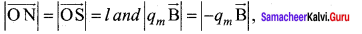The magnitude of total torque about point O τ = l × qm B sin θ +l × qm B sin θ τ = 2l xqm B sin θ τ = Pm B sin θ (∴ qm × 2l = Pm ) In vector notation, τ = pm × B⃗

Question 5. Calculate the magnetic induction at a point on the a×ial line of a bar magnet.
Answer: Magnetic field at a point along the axial line of the magnetic dipole (bar magnet): Consider a bar magnet NS. Let N be the North Pole and S be the south pole of the bar magnet, each of pole strength qm and separated by a distance of 2l. The magnetic field at a point C (lies along the axis of the magnet) at a distance from the geometrical center O of the bar magnet can be computed by keeping unit north pole (qMC = 1 A m) at C. The force experienced by the unit north pole at C due to pole strength can be computed using Coulomb’s law of magnetism as follows: The force of repulsion between north pole of the bar magnet and unit north pole at point C (in free space) is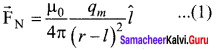where r – l is the distance between north pole of the bar magnet and unit north pole at C. The force of attraction between South Pole of the bar magnet and unit North Pole at point C (in free space) is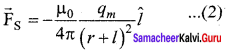where r + 1 is the distance between south pole of the bar magnet and unit north pole at C.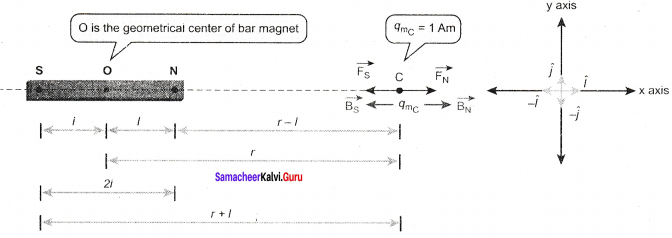From equation (1) and (2), the net force at point C is F⃗  = F⃗ N + F⃗ S. From definition, this net force is the magnetic field due to magnetic dipole at a point C(F⃗  = B⃗ )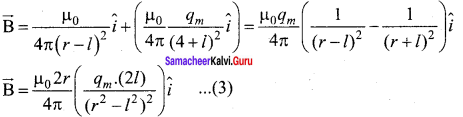Since, magnitude of magnetic dipole moment is ∣∣P⃗ m∣∣ pm = qm. 2l the magnetic field point C equation (3) can be written as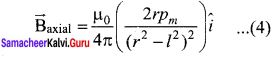If the distance between two poles in a bar magnet are small (looks like short magnet) compared to the distance between geometrical centre O of bar magnet and the location of point C i.e., r >>1 then, (r2 – l2)2 ≈ r4 ….. (5) Therefore, using equation (5) in equation (4), we get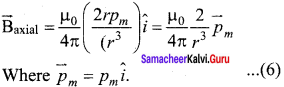Question 6. Obtain the magnetic induction at a point on the equatorial line of a bar magnet. Magnetic field at a point along the equatorial line due to a magnetic dipole (bar magnet)
Answer: Consider a bar magnet NS. Let N be the north pole and S be the south pole of the bar magnet, each with pole strength qm and separated by a distance of 2l. The magnetic field at a point C (lies along the equatorial line) at a distance r from the geometrical center O of the bar magnet can be computed by keeping unit north pole (qmC = 1 A m) at C. The force experienced by the unit north pole at C due to pole strength N-S can be computed using Coulomb’s law of magnetism as follow’s: The force of repulsion between North Pole of the bar magnet and unit north pole at point C (in free space) is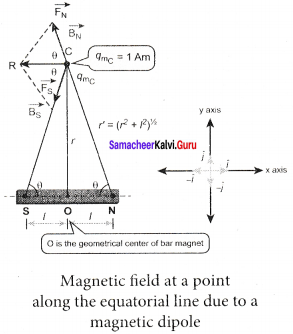F⃗ N = -FN cos θ i^ + FN sin θ j^ …… (1) Where FN = µ04π qmr′2 The force of attraction (in free space) between south pole of the bar magnet and unit north pole at point C is F⃗ S = -FS cos θ i^ + FS sin θ j …… (2) Where F⃗ S = µ04π qmr′2 From equation (1) and equation (2), the net force at point C is F⃗  = FN + FS This net force is equal to the magnetic field at the point C. B⃗  = -(FN + FS) cos θ i^ Since, FN = FS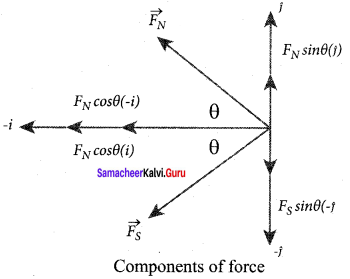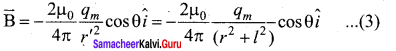In a right angle triangle NOC as shown in the Figure 1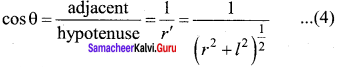Substituting equation 4 in equation 3 We get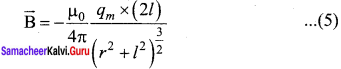Since, magnitude of magnetic dipole moment is ∣∣P⃗ m∣∣ = Pm = qm. 2l and substituting in equation (5), the magnetic field at a point C is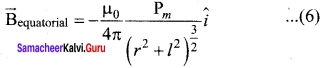If the distance between two poles in a bar magnet are small (looks like short magnet) when compared to the distance between geometrical center O of bar magnet and the location of point C i.e., r>> l, then, (r2 + l2)32 Therefore, using equation (7) in equation (6), we get ≈ r3 ……… (7)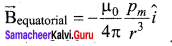Since Pm i^ = ∣∣P⃗ m∣∣m, in general, the magnetic field at equatorial point is given by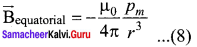Note that magnitude of Baxial is twice that of magnitude of Bequatorial and the direction of are opposite.

Question 7. Find the magnetic induction due to a long straight conductor using Ampere’s circuital law. Magnetic field due to the current carrying wire of infinite
Answer: length using Ampere’s law: Consider a straight conductor of infinite length carrying current I and the direction of magnetic field lines. Since the wire is geometrically cylindrical in shape C and symmetrical about its axis, we construct an Amperian loop in the form of a circular shape at a distance r from the centre of the conductor. From the Ampere’s law, we get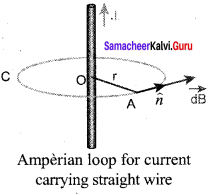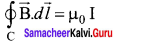Where dl is the line element along the amperian loop (tangent to the circular loop). Hence, the angle between magnetic field vector and line element is zero. Therefore,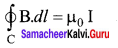where I is the current enclosed by the Amperian loop. Due to the symmetry, the magnitude of the magnetic field is uniform over the Amperian loop, we can take B out of the integration.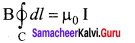For a circular loop, the circumference is 2πr, which implies,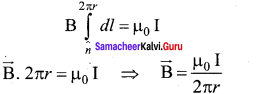In vector form, the magnetic field is B⃗  = μ0I2πrn^ Where n^ is the unit vector along the tangent to the Amperian loop. This perfectly agrees with the result obtained from Biot-Savarf s law as given in equation B⃗  = μ0I2πan^

Question 8. Discuss the working of cyclotron in detail.
Answer: Cyclotron: Cyclotron is a device used to accelerate the charged particles to gain large kinetic energy. It is also called as high energy accelerator. It was invented by Lawrence and Livingston in 1934.

Principle: When a charged particle moves normal to the magnetic field, it experiences magnetic Lorentz force.

Construction: The particles are allowed to move in between two semicircular metal containers called Dees (hollow D – shaped objects). Dees are enclosed in an evacuated chamber and it is kept in a region with uniform magnetic field controlled by an electromagnet. The direction of magnetic field is normal to the plane of the Dees. The two Dees are kept separated with a gap and the source S (which ejects the particle to be accelerated) is placed at the center in the gap between the Dees. Dees are connected to high frequency alternating potential difference.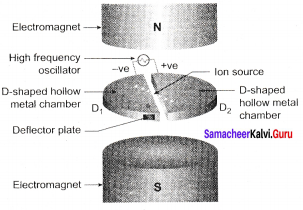Working: Let us assume that the ion ejected from source S is positively charged. As soon as ion is ejected, it is accelerated towards a Dee (say, Dee – 1) which has negative potential at that time. Since the magnetic field is normal to the plane of the Dees, the ion undergoes circular path. After one semi-circular path in Dee-1, the ion reaches the gap between Dees. At this time, the polarities of the Dees are reversed so that the ion is now accelerated towards Dee-2 with a greater velocity. For this circular motion, the centripetal force of the charged particle q is provided by Lorentz force. mv2r qvB ⇒ r = mqb v ⇒ r ∝ v From the equation, the increase in velocity increases the radius of circular path. This process continues and hence the particle undergoes spiral path of increasing radius. Once it reaches near the edge, it is taken out with the help of deflector plate and allowed to hit the target T. Very important condition in cyclotron operation is the resonance condition. It happens when the frequency ƒ at which the positive ion circulates in the magnetic field must be equal to the constant frequency of the electrical oscillator fosc From equation fosc = qB2πm T = 1fosc The time period of oscillation is T = 2πmqB The kinetic energy of the charged paricle is K E = 12 mv2 = q2B2r22m

Limitations of cyclotron: (a) the speed of the ion is limited (b) electron cannot be accelerated (c) uncharged particles cannot be accelerated

Question 9. What is tangent law? Discuss in detail.
Answer: Tangent law: Statement: When a magnetic needle or magnet is freely suspended in two mutually perpendicular uniform magnetic fields, it will come to rest in the direction of the resultant of the two fields.

Explanation: Let B be the magnetic field produced by passing current through the coil of the tangent Galvanometer and BH be the horizontal component of earth’s magnetic field. Under the action of two magnetic fields, the needle comes to rest making angle θ with BH, such that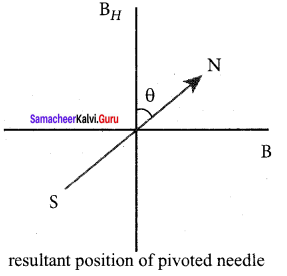tan θ = BBH B = BH tan θ ……. (1) When no current is passed through the coil, the small magnetic needle lies along horizontal component of Earth’s magnetic field. When the circuit is switched ON, the electric current will pass through the circular coil and produce magnetic field. Now there are two fields which are acting mutually perpendicular to each other. They are: (1) the magnetic field (B) due to the electric current in the coil acting normal to the plane of the coil. (2) the horizontal component of Earth’s magnetic field (BH) Because of these crossed fields, the pivoted magnetic needle deflects through an angle θ. From tangent law, B = BH tan θ When an electric current is passed through a circular coil of radius R having N turns, the magnitude of magnetic field at the center is B = µ0 NI2R …….. (2) From equation (1) and equation (2), we get µ0 NI2R = BH tan θ The horizontal component of Earth’s magnetic field can be determined as B = µ0 NI2Rtanθ in tesla ……. (3)

Question 10. Explain the principle and working of a moving coil galvanometer.
Answer: Moving coil galvanometer: Moving coil galvanometer is a device which is used to indicate the flow of current in an electrical circuit.

Principle: When a current carrying loop is placed in a uniform magnetic field it experiences a torque.

Construction: A moving coil galvanometer consists of a rectangular coil PQRS of insulated thin copper wire. The coil contains a large number of turns wound over a light metallic frame. A cylindrical soft-iron core is placed symmetrically inside the coil. The rectangular coil is suspended freely between two pole pieces of a horse-shoe magnet.

The upper end of the rectangular coil is attached to one end of fine strip of phosphor bronze and the lower end of the coil is connected to a hair spring which is also made up of phosphor bronze. deflection of the coil with the help of lamp and scale arrangement. The other end of the mirror is connected to a torsion head T. In order to pass electric current through the galvanometer, the suspension strip W and the spring S are connected to terminals.

Working: Consider a single turn of the rectangular coil PQRS whose length be l and breadth b. PQ = RS = l and QR = SP = b. Let I be the electric current flowing through the rectangular coil PQRS. The horse-shoe magnet has hemi – spherical magnetic poles which produces a radial magnetic field.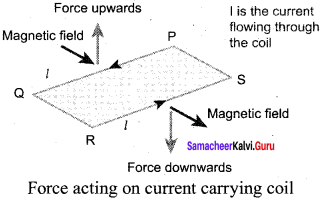Due to this radial field, the sides QR and SP are always parallel to to the B-field (magnetic field) and experience no force. The sides PQ and RS are always parallel to the B-field and experience force and due to this, torque is produced. For single turn, the deflection couple. τ = bF = bBIl= (lb) BI = ABI since, area of the coil A = lb For coil with N turns, we get r = NABI …… (1) Due to this deflecting torque, the coil gets twisted and restoring torque (also known as restoring couple) is developed. Hence the magnitude of restoring couple is proportional to the amount of twist θ. Thus τ = K θ ……. (2) where K is the restoring couple per unit twist or torsional constant of the spring. At equilibrium, the deflection couple is equal to the restoring couple. Therefore by comparing equation (1) and (2), we get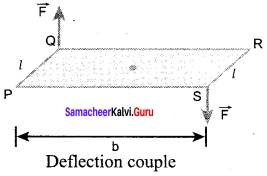NABI = Kθ ⇒ I = KNABθ …….. (3) (or) I = G θ Where, G = KNAB is called is called galvanometer constant or current reduction factor of the galvanometer. Since, suspended moving coil galvanometer is very sensitive, we have to handle with high care while doing experiments. Most of the galvanometer we use arc pointer type moving coil galvanometer.

Question 11. Discuss the conversion of galvanometer into an ammeter and also a voltmeter.
Answer: Conversion of galvanometer into ammeter and voltmeter: A galvanometer is very sensitive instrument to detect the current. It can be easily converted into ammeter and voltmeter. (i) Galvanometer to an Ammeter: Ammeter is an instrument used to measure current flowing in the electrical circuit. The ammeter must offer low resistance such that it will not change the current passing through it. So ammeter is connected in series to measure the circuit current.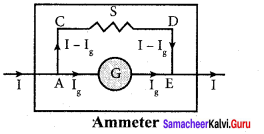A galvanometer is converted into an ammeter by connecting a low resistance in parallel with the galvanometer. This low resistance is called shunt resistance S. The scale is now calibrated in ampere and the range of ammeter depends on the values of the shunt resistance.

Let I be the current passing through the circuit. When current I reaches the junction A, it divides into two components. Let Ig be the current passing through the galvanometer of resistance Rg through a path AGE and the remaining current (I – Ig) passes along the path ACDE through shunt resistance S.

The value of shunt resistance is so adjusted that current I produces full scale deflection in the galvanometer. The potential difference across galvanometer is same as the potential difference across shunt resistance.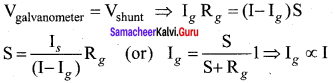Since, the deflection in the galvanometer is proportional to the current passing through it. θ = 1g Ig ⇒ θ Ig ⇒ θ ∝ I So, the deflection in the galvanometer measures the current I passing through the circuit (ammeter). Shunt resistance is connected in parallel to galvanometer. Therefore, resistance of ammeter can be determined by computing the effective resistance, which is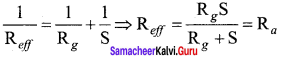Since, the shunt resistance is a very low resistance and the ratio SRg is also small. This means, Rg is also small, i.e., the resistance offered by the ammeter is small. So, when we connect ammeter in series, the ammeter will not change the resistance appreciably and also the current in the circuit. For an ideal ammeter, the resistance must be equal to zero. Hence, the reading in ammeter is always lesser than the actual current in the circuit. Let Iideal be current measured from ideal ammeter and Iactual be the actual current measured in the circuit by the ammeter. Then, the percentage error in measuring a current through an ammeter is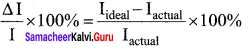(ii) Galvanometer to a voltmeter: A voltmeter is an instrument used to measure potential difference across any two points in the electrical circuits. It should not draw any current from the circuit otherwise the value of potential difference to be measured will change. Voltmeter must have high resistance and when it is connected in parallel, it will not draw appreciable current so that it will indicate the true potential difference.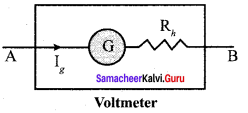A galvanometer is converted into a voltmeter by connecting high resistance Rh in series with galvanometer. The scale is now calibrated in volt and the range of voltmeter depends on the values of the resistance connected in series i.e. the value of resistance is so adjusted that only current Ig produces full scale deflection i nthe galvanometer.

Let Rg be the resistance of galvanometer and ‘g be the current with which the galvanometer produces full scale deflection. Since the galvanometer is connected in series with high resistance, the current in the electrical circuit is same as the current passing through the galvanometer. Potential difference I = Ig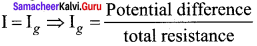Since the galvanometer and high resistance are connected in series, the total resistance or effective resistance gives the resistance of voltmeter. The voltmeter resistance is Rv Rg + Rh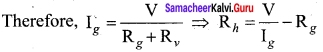Note that Ig ∝ V The deflection in the galvanometer is proportional to current I . But current is proportional to the potential difference. Hence the deflection in the ga’anometer is proportional to potential difference. Since the resistance of voltmeter is very large, a voltmeter connected in an electrical circuit will draw least current in the circuit. An ideal voltmeter is one which has infinite resistance.

Question 12. Calculate the magnetic field inside and outside of the long solenoid using Ampere’s circuital law.
Answer: Magnetic field due to a long current carrying solenoid: Consider a solenoid of length L having N turns. The diametre of the solenoid is assumed to be much smaller when compared to its length and the coil is wound very closely.

In order to calculate the magnetic field at any point inside the solenoid, we use Ampere’s circuital law. Consider a rectangular loop abed. Then from Ampere’s circuital law,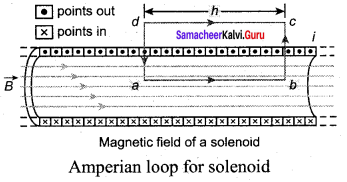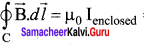= µ0 x (total current enclosed by Amperian loop) The left hand side of the equation is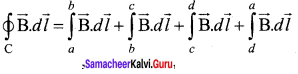Since the elemental lengths along bc and da are perpendicular to the magnetic field which is along the axis of the solenoid, the integrals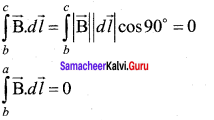Since the magnetic field outside the solenoid is zero, the integral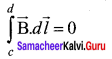For the path along ab, the integral is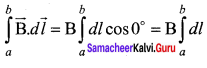where the length of the loop ab is h. But the choice of length of the loop ab is arbitrary. We ean take very large loop such that it is equal to the length of the solenoid L. Therefore the integral is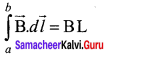let N I be the current passing through the solenoid of N turns, then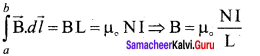The number of turns per unit length is given by NIL = n, then B = µ0 nLIL = µ0nI Since n is a constant for a given solenoid and p0 is also constant. For a fixed current I, the magnetic field inside the solenoid is also a constant.

Magnetism and Magnetic Effects of Electric Current Numerical problems

Question 1. A bar magnet having a magnetic moment M−→ is cut into four pieces i.e., first cut in two pieces along the axis of the magnet and each piece is further cut into two pieces. Compute the magnetic moment of each piece. Solution: Consider a bar magnet of magnetic moment M−→ . When a bar magnet first cut in two pieces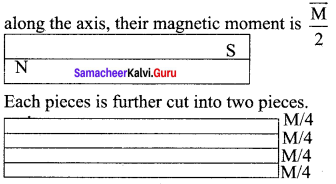Their magnetic moment of each pieces M−→4 Their magnetic moment of each pieces M−→new 14 M−→

Question 2. A conductor of linear mass density 0.2 g m-1 suspended by two flexible wire as shown in figure. Suppose the tension in the supporting wires ¡s zero when it is kept inside the magnetic field of I T whose direction is into the page. Compute the current inside the conductor and also the direction of the current. Assume g = 10 m s-2.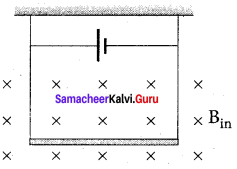Solution: Downward force, F = mg Linear mass density, ml = 0.2 gm-1 ml = 0.2 x 10-3 kg m-1 m = (0.2 x 10-3 x l) kg m-1 F = (0.2 x 10-3 x l x 10) N Upward magnetic force acting on the wire F = BIl 0.2 x 10-3 x l x 10 = 1 x I x l I = 2 x 10-3 I = 2 m A

Question 3. A circular coil with cross-sectional area 0.1 cm2 is kept in a uniform magnetic field of strength 0.2 T. If the current passing in the coil is 3 A and plane of the loop is perpendicular to the direction of magnetic field. Calculate (a) total torque on the coil (b) total force on the coil (c) average force on each electron in the coil due to the magnetic field of the free electron density for the material of the wire is 1028 m-3 Solution: Cross sectional area of coil, A = 0.1 cm2 A = 0.1 x 10-4m2 Uniform magnetic field of strength, B = 0.2T Current passing in the coil, I = 3A Angle between the magnetic field and normal to the coil, θ = 0° (a) Total torque on the coil, τ = ABI sin θ = 0.1 x 10-4 x 0.2 x 3 sin0° sin0° = 0 τ = 0

(b) Total force on the coil F = BIl sin θ = 0.2 x 3 x l x sin 0° F = 0

(c) Average force: F = qVdB drift velocity, Vd = 1neA [∵ q = e] F = e (1neA)B [∵n = 1028 m-3 IBnA = 3×0.21028×0.1×10−4 = 6 x 10-24 Fav = 0.6 x 10-23 N

Question 4. A bar magnet is placed in a uniform magnetic field whose strength is 0.8 T. Suppose the bar magnet orient at an angle 30° with the external field experiences a torque of 0.2 N m. Calculate: (i) the magnetic moment of the magnet (ii) the work done by an applied force in moving it from most stable configuration to the most unstable configuration and also compute the work done by the applied magnetic field in this case. Solution: Uniform magnetic field strength B = 0.8T Bar magnet orient an angle with magnetic field θ = 30° Torque τ = 0.2 Nm (i) Magnetic moment of the magnet, Torque τ = Pm B Sin θ ∴ Magnetic moment, Pm = τBsinθ = 0.20.8×Sin30° = 0.20.4 Pm = 0.5 Am2

(ii) Work done by external torque is stored in the magnet as potential energy. W = U = – Pm B Sin θ Here, applied force acting on magnet its moving from most stable θ’ to most unstable θ. θ’= 0° and θ = 180° So, workdone W = U = – Pm B (Cosθ – Cosθ’) = – Pm B (Cos 180° – Cos 0°) = – 0.5 x 0.8 ((-1) – 1) = – 0.4 (-2) W = U = 0.8 W= 0.8 J

Question 5. A non – conducting sphere has a mass of 100 g and radius 20 cm. A flat compact coil of wire with turns 5 is wrapped tightly around it with each turns concentric with the sphere. This sphere is placed on an inclined plane such that plane of coil is parallel to the inclined plane. A uniform magnetic field of 0.5 T exists in the region in vertically upward direction. Compute the current 1 required to rest the sphere in equilibrium.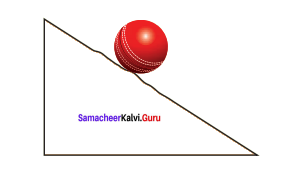Solution: At equilibrium fs R-pm B sin θ = 0 mgR = NBAI I = mgRNBA = mgRNBπR2 I = mgπRNB Mass of the sphere, m= 100 g = 100 x 10-3 kg Radius of the sphere R = 20 cm = 20 x 10-2 m Number of turns n = 5 Uniform magnetic field B = 0.5 T I = 100×10−3×10π×20×10−2×5×0.5 = 1000×10−3π×50×10−2 = 20×10−1π I = 2π A.

Question 6. Calculate the magnetic field at the center of a square loop which carries a current of 1.5 A, length of each loop is 50 cm. Solution: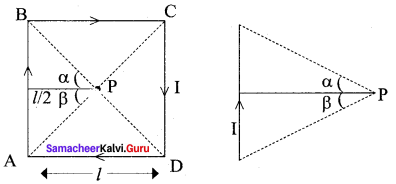Current through the square loop, I = 1.5A Length of each loop, l = 50cm 50 x 10-2 m According to Biot-Savart Law. Magnetic field due to a current carrying straight wire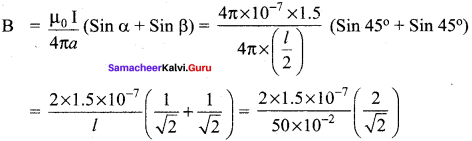B =0.084866 x 10-5 T Magnetic field at a point p? of centre of current carrying square ioop B’ =4 sides x B = 4 x 0.08487 x 10-5 = 0.33948 x 10-5 B’ =3.4 x 10-6 T

Question 7. Show that the magnetic field at any point on the axis of the solenoid having n turns per unit length is B = 12 µ0 nI (cos θ1 – cosθ2) Solution: A solenoid is a cylindrical coil having number of circular turns. Consider a solenoid having radius R consists of n number’of turns per unit length. Let ‘P’ be the point at a distance ‘x’ from the origin of the solenoid. The current carrying element dx at a distance x from origin and the distance r from point ‘P’. r = R2+(x0−x)2−−−−−−−−−−−−√ The magnetic field due to current carrying circular coil at any axis is dB = μ0IR22r3 × N Where N = ndx, then dB = µ02 nIR2dxr3 ……. (1) sin θ = Rr r = R × 1sinθ = R cosec θ ……. (2) tan θ = Rx0−x x0 – x = R × 1tanθ = R cot θ dxdθ = R cosec2 θ ⇒ dx = R cosec2 θ dθ ……. (3) From above three equations, wer get dB = µ02 nIR2(Rcsc2θdθ)R3csc3θ dB = µ02 n I sin θ dθ Now total magnetic field can be obtained by integrating from θ1 to θ2, we get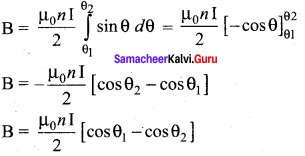Question 8. Let I1 and I2 be the steady currents passing through a long horizontal wire XY and PQ respectively. The wire PQ is fixed in horizontal plane and the wire XY be is allowed to move freely in a vertical plane. Let the wire XY is in equilibrium at a height d over the parallel wire PQ as shown in figure.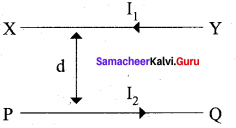Solution: If the wire XY is slightly displaced and released, it executes simple uarmonic motion due to force of repulsion produced between the current carrying wire. Acceleration of the wire, a = -ω2y Time period of oscillation of the wire, T = 2π dg−−√

### Samacheer Kalvi 12th Physics Magnetism and Magnetic Effects of Electric Current Additional Questions Solved

I. Choose the Correct

Question 1. Source (s) of a magnetic field is (are)- (a) an isolated magnetic pole (b) a static electric charge (c) a moving electric charge (d) all of these
Answer: (c) a moving electric charge

Question 2. Magnetic field lines- (a) cannot intersect at all (b) intersect at infinity (c) intersect within the magnet (d) intersect at the neutral points
Answer: (a) cannot intersect at all

Question 3. A magnetic needle is kept in a non-uniform magnetic field. It experiences- (a) a force and a torque (b) a force but not a torque (c) a torque but not a force (d) neither a force nor a torque
Answer: (a) a force and a torque

Question 4. The SI unit of pole strength is- (a) Am2 (b) Am-1 (c) Am-2 (d) Am

Question 5. Earth’s magnetic field always has a horizontal component except at- (a) equator (b) magnetic pole (c) a latitude of 60° (d) a latitude of 50°

Question 6. The angle of dip at the magnetic equator is- (a) 0° (b) 30° (c) 60° (d) 90°

Question 7. At a certain place the horizontal component of earth’s magnetic field is √3 times vertical component. The angle of dip at that place is- (a) 75° (b) 60° (c) 45° (d) 30°
Answer: (d) 30° Hint: tan δ = BVBH = 1√3 ⇒ δ = tan-1 (13√) = 30°

Question 8. At magnetic poles the angle of dip is- (a) 45° (b) 30° (c) 0° (d) 90°

Question 9. The horizontal component of earth’s magnetic field at a place is 3.6 x 10-5T. If the angle of dip at this place is 60°. the vertical components of earth’s field at this place is- (a) 1.2 x 10-5T (b) 2.4 x 10-5T (c) 4 x 10-5T (d) 6.2 x 10-5T
Answer: (d) 6.2 x 10-5T Hint: Bv = BH tan δ = 3.6 x 10-5 x tan 60° Bv = 6.2 x 10-5T

Question 10. A bar magnet of magnetic moment M is cut into two parts of equal length. The magnetic moment of either part is- (a) M (b) 2M (c) M2 (d) Zero

Question 11. A magnetic needle suspended by a silk thread is vibrating in the earths magnetic field, If the temperature of the needle is increased by 500°C, then- (a) time period decreases (b) time period remains unchanged (c) time period increases (d) the needle stops vibrating
Answer: (c) time period increases Hint: Magnet moment decreases with temperature. Therefore the time period will increase.

Question 12. Demagnetisation of a magnet can be done by- (a) rough handling (b) magnetising in the opposite direction (c) heating (d) all the above

Question 13. All the magnetic materials lose their magnetic properties when- (a) dipped in water (b) dipped in oil (c) brought near a piece of iron (d) strongly heated

Question 14. The relative permeability of a paramagnetic material is- (a) greater than unity (b) less than unity (c) equal to unity (d) negative

Question 15. The relative permeability of a diamagnetic material is- (a) greater than unity (b) less than unity (c) equal to unity (d) negative

Question 16. Which of the following is most suitable for the core of an electromagnet? (a) air (b) soft iron (c) steel (d) Co-Ni alloy

Question 17. Soft Iron is used in many parts of electrical machines for- (a) low hysteresis loss and low permeability (b) low hysteresis less and high permeability (c) high hysteresis loss and low permeability (d) high hysteresis loss and high permeability
Answer: (b) low hysteresis loss and high permeability

Question 18. When a diamagnetic substance is brought near the north or the south pole of a bar magnet, it is- (a) attracted by the poles (b)repel led by the poles (c) attracted by the north pole and repelled by the south pole (d) attracted by the south pole and repelled by the north pole
Answer: (b) repelled by the poles

Question 19. At Curie point, a ferromagnetic material becomes- (a) non magnetic (b) diamagnetic (c) paramagnetic (d) antiferromagnetic

Question 20. Magnetic permeability is maximum for- (a) diamagnetic substances (b) paramagnetic substances (c) ferromagnetic substances (d) all of these

Question 21. The material of a permanent magnet has- (a) high retentivity, low coercivity (b) low retentivity, low coercivity (c) low retentivity, low coercivity (d) high retentivity, high eoercivity
Answer: (d) high retentivity, high cuercivity

Question 22. Which one of the following is not made of soft iron? (a) electromagnet (b) core of transformer (c) core of dynamo (d) magnet of loudspeaker

Question 23. A dip circle k at right angles to the magnetic meridian. The apparent dip is- (a) 0° (b) 30° (c) 600 (d) 90°

Question 24. A magnetic needle is placed in a uniform magnetic field. It experience- (a) a force and a torque (b) a force but not a torque (c) a torque but not a force (d) neither a force nor a torque
Answer: (c) a torque but not a force

Question 25. Relative permeability of iron is 5500. Its magnetic susceptibility is- (a) 5501 (b) 5500 x 10-7 (c) 5500 x 107 (d) 5499
Answer: (d) 5499 Hint: µr = 1 + x ⇒ x = µr -1 = 5500 – 1 = 5499

Question 26. The inherent property of all matter is / are- (a) paramagnetism (b) diamagnetism (c) ferromagnetism (d) all the above

Question 27. A magnetic needle suspended freely- (a) orients itself in a definite direction (b) remains in any direction (c) become vertical with N-pole up (d) become vertical with N-pole down
Answer: (a) orients itself in a definite direction

Question 28. The earth’s magnetic field at a given point is 0.5 x10-5 Wb/m2. This field is to be annulled by magnetic induction at the center of circular conducting loop of radius 5 cm. The current required to be flown in the loop is nearly- (a) 0.2 A (b) 0.4 A (c) 4 A (d) 40 A
Answer: (b) 0.4 A Hint: B = µ0I2r ⇒ I = 2rBµ0 = 2×0.05×0.5×10−54π×10−7 = 04 A

Question 29. A frog can be leviated in a magnetic field produced by a current in a vertical solenoid placed below the frog. This is possible because the body of the frog is- (a) paramagnetic (b) diamagnetic (c) ferromagnetic (d) antiferromagnetic

Question 30. The magnetic moment of a current carrying circular coil of ladius r varies as- (a) 1r2 (b) 1r (c) r (d) r2
Answer: (d) r2 Hint: Magnetic moment, M = 1A = I(πr2) = M αr2

Question 31. For a paramagnetic material, the dependence of the magnetic susceptibility % on the absolute temperature T is given as- (a) x ∝ r (b) x ∝ 1T2 (c) x ∝ 1T (d) x ∝ T2

Question 32. A charged particle (charge q) is moving in a circle of radius R with uniform speed V. The associated magnetic moment is given by- (a) qVR2 (b) qVR22 (c) qVR (d) qVR2
Answer: (d) qVR2 Hint: Magnetic moment, M = IA = qT (π R2) M = qv2πR (π R2) = qvR2

Question 33. Nickel shows ferromagnetic property at room temperature. If the temperature is increased . beyond Curie temperature, then it will show- (a) antiferromagnetism (b) no magnetic property (c) diamagnetism (d) para magnetism

Question 34. Aproton enters a magnetic field of flux density 1.5 Wb/m2 with a speed of 2x 107 m/s at angle of 30° with the field. The force on the proton will be- (a) 0.24 x 10-12 N (b) 2.4 x 10-12 N (c) 24 x 10-12 N (d) 0.024 x 10-12 N
Answer: (b) 2.4 x 10-12 N Hint: F = Bqv sin θ =1.5 x 1.6 x 10-19 x 2 x 107 x sin 30° = 2.4 x 10-12 N

Question 35. A moving charge produces- (a) an electric field only (b)a magnetic field only (c) both electric and magnetic fields (d) neither an electric nor a magnetic field
Answer: (c) both electric and magnetic fields

Question 36. A straight conductor carrying a current I, is split into a circular loop of radius r as shown in the figure. The magnetic field at the centre O of the circle, in tesla is-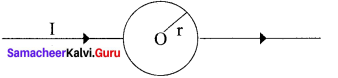(a) µ0I2r (b) µ0I2πr (c) µ0Iπr (d) zero
Answer: (d) zero Hint: Field due to the upper and lower semicircles will cancel out.

Question 37. In a moving coil galvanometer the current ‘i’ is related to the deflection θ as- (a) i α θ (b) i α tan θ (c) i α θ2 (d) i α √θ

Question 38. The magnetic field due to a current carrying circular coil on the axis, at a large distance r from the centre of the coil, varies approximately as- (a) 1r (b) 1r32 (c) 1r3 (d) 1r2

Question 39. A moving charge is subjected to an external magnetic field. The change in the kinetic energy of the particle- (а) increases with the increase in the field strength (b) decreases with the increase in the field strength (c) is always zero (d) depends upon whether the field is uniform or non-uniform

Question 40. Lorentz force is given by- (a) q (E + V × B) (b)q (E -V × B) (c) q (E + V.B) (d) q (E × B × V)
Answer: (a) q (E + V × B)

Question 41. A circular loop has radius R and a current I flows through it. Another circular loop has radius 2R and a current 21 flows through it. Ratio of the magnetic fields at their centres is- (a) 14 (b) 1 (c) 2 (d) 4
Answer: (b) 1 Hint: B1 = µ0I2r and B2 = µ(2I)2(2R) ; B1B2 = 1

Question 42. The magnetic field inside a solenoid is- (a) directly proportional to current (b) inversely proportional to current (c) directly proportional to its length (d) inversely proportional to the total number of turns
Answer: (a) directly proportional to current

Question 43. A circular loop of area 0.01 m2 and carrying a current of 10A is placed parallel to a magnetic field of intensity 0.1T. The torque acting on the loop, in Nm is- (a) 1.1 (b) 0.8 (c) 0.001 (d) 0.01
Answer: (d) 0.01 Hint: τ = BIA = 0.1 x 10 x 0.01 = 0.01 Nm

Question 44. In a current carrying long solenoid the field produced does not depend upon- (a) number of turns per unit length (b) current flowing (c) radius of the solenoid (d) all of the above

Question 45. When a charged particle enters a uniform magnetic field its kinetic energy- (a) remains constant (b) increases (c) decreases (d) becomes zero

II Fill in the blanks

Question 1. At Curie point, a ferromagnetic material becomes ………………

Question 2. Electromagnets are made of soft iron because soft iron has ………………
Answer: high susceptibility and low retentivity

Question 3. The word magnetism is derived from iron ore ………………

Question 4. The chemical formula of magneties is ………………

Question 5. The iron ore magnetite was found in the island of ………………

Question 6. ……………… suggested that earth behaves as a giant bar magnet

Question 7. The field at the surface of the earth is approximately equal to

Question 8. The natural magnets have ………………
Answer: irregular shape and they are weak

Question 9. Pieces of iron or steel that acquires magnetic properties when it is rubbed with a magnet are called ………………

Question 10. Artificial magnet in the form of a rectangular or cylinderical bar is called ………………

Question 11. In magnet, the magnetic attraction is maximum at the ……………… of the magnet.

Question 12. The unit of pole strength is ………………

Question 13. The unit of magnetic flux density is ………………

Question 14. The value of po is equal to ………………

Question 15. A unit pole experiences a force of ………………

Question 16. At neutral points the resultant magnetic field due to the magnet and earth is ………………
Answer: magnetic field of the earth

Question 17. The circular scale of deflection magneto meter is divided into ……………… quadrants?

Question 18. Each quadrant of the circular scale of deflection magnetometer is graduated from ………………

Question 19. The sensitivity of the deflection magneto meter is more at ………………

Question 20. The magnetic field used to magnetise a material is called the ………………

Question 21. The unit of magnetising field or magnetic intensity is ………………

Question 22. The pole strength per unit area of the cross-section of the material is termed as

Question 23. The magnetic moment per unit volume of the material is termed as ………………

Question 24. In diamagnetic materials the net magnetic moment of atoms is ………………

Question 25. The susceptibility of diamagnetic materials has a ……………… value.

Question 26. The susceptibility (xm) of bismuth is ………………

Question 27. The relative permeability of diamagnetic material is ………………

Question 28. Ferromagnetic substances have ………………magnetic moment.

Question 29. As the temperature increase, the value of susceptibility of the ferromagnetic substance ………………

Question 30. The phenomenon of lagging of magnetic induction behind the magnetising field is called ………………

Question 31. The direction of the magnetic field in a current carrying conductor is given by ………………
Answer: Maxwell’s right cork screw rule

Question 32. The relative permeability (µr) for air is ………………

Question 33. The instrument used for measuring current is ………………

Question 34. Tangent galvanometer works on the principle of ………………

Question 35. The tangent law is applied in ………………

III Match the following

Question 1.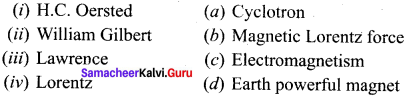Answer: (i) → (c) (ii) → (d) (iii) → (a) (iv) → (b)

Question 2.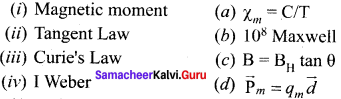Answer: (i) → (c) (ii) → (d) (iii) → (b) (iv) → (a)

Question 3.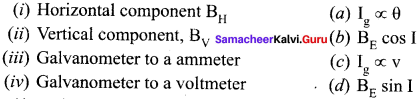Answer: (i) → (c) (ii) → (a) (iii) → (d) (iv) → (b)

Question 4.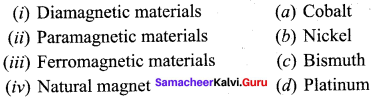(i) → (d) (ii) → (c) (in) →(a) (iv) → (b)

Question 5.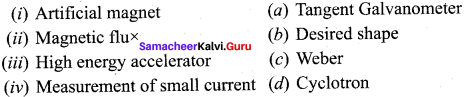Answer: (i) → (d) (ii) → (a) (iii) → (b) (iv) → (c)

IV Assertion and Reason Questions

(a) If both assertion and reason are true and reason is the correct explanation of assertion. (b) If both assertion and reason are true but reason is not the correct explanation of assertion. (c) If assertion is true but reason is false. (d) If assertion is false but reason is true. (e) If both assertion and reason are false.

Question 1. Assertion: The poles of magnet cannot be separated by breaking into two pieces. Reason: The magnetic moment will be reduced to half when a magnet is broken into two equal pieces.
Answer: (b) If both assertion and reason are true but reason is not the correct explanation of assertion. Explanation: As we know every atom of a magnet acts as a dipole, so poles cannot be separated. When magnet is broken into two equal pieces, magnetic moment of each part will be half of the original magnet.

Question 2. Assertion: When radius of circular loop carrying current is doubled, its magnetic moment becomes four times. Reason: Magnetic moment depends on area of the loop.
Answer: (b) If both assertion and reason are true but reason is not the correct explanation of assertion. Explanation: Magnetic moment M = IA = I (πr2) New magnetic moment Ml = I π (2r)2 = 4πIr2 = 4M

Question 3. Assertion: The ferromagnetic substance do not obey Curie’s Law. Reason: At Curie point a ferromagnetic substance start behaving as a paramagnetic substance.
Answer: (b) If both assertion and reason are true but reason is not the correct explanation of assertion. Explanation: The susceptibility of ferromagnetic substance decreases with the rise of temperature in a complicated manner. After Curie point the susceptibility ferromagnetic substance varies inversely with its absolute temperature.

Question 4. Assertion: Soft iron is used as transformer core. Reason: Soft iron has narrow hysteresis loop.
Answer: (a) If both assertion and reason are true and reason is the correct explanation of assertion. Explanation: For high efficiency of transformer, the energy loss will be lesser if the hysteresis loop is lesser area, i.e narrow.

Question 5. Assertion: Cyclotron does not accelerate electron. Reason: Mass of the electron is very small
Answer: (a) If both assertion and reason are true and reason is the correct explanation of assertion. Explanation: Cyclotron is suitable for accelerating heavy particles like protons, x-particles. etc. and not for electrons because of low mass.

Question 6. Assertion: Cyclotron is a device which is used to accelerate the positive ion. Reason: Cyclotron frequency depends upon the velocity.
Answer: (c) If assertion is true but reason is false. Explanation: Cyclotron is utilised to accelerate the positive ion. And cyclotron frequency is given by v =Be2πm. It means cyclotron frequency doesn’t depends upon velocity.

Magnetism and Magnetic Effects of Electric Current 2-mark Questions

Question 1. Define magnetic declination (D).
Answer: The angle between magnetic meridian at a point and geographical meridian is called the declination or magnetic declination (D).

Question 2. Define magnetic inclination (I).
Answer: The angle subtended by the Earth’s total magnetic field B with the horizontal direction in the magnetic meridian is called dip or magnetic inclination (I) at the point.

Question 3. Define magnetic field.
Answer: The magnetic field B⃗  at a point is defined as a force experienced by the bar magnet of unit pole strength. B = 1qm F⃗  Its unit is N A-1 m-1

Question 4. Define magnetic flux density.
Answer: The magnetic flux density is defined as the number of magnetic field lines crossing unit area kept normal to the direction of line of force. Its unit is Wb m-2 or tesla.

Question 5. State ‘Tangent Law’.
Answer: When a magnetic needle or magnet is freely suspended in two mutually perpendicular uniform magnetic fields, it will come to rest in the direction of the resultant of the two fields.

Question 6. Define in terms of magnetising field.
Answer: The magnetic field which is used to magnetize a sample or specimen is called the magnetising field. Magnetising field is a vector quantity and it denoted by H⃗  and its unit is A m-1 .

Question 7. State Right hand thumb rule.
Answer: If we curl the fingers of right hand in the direction of current in the loop, then the stretched thumb gives the direction of the mag netic moment associated with the loop.

Question 8. State ‘One ampere’.
Answer: One ampere is defined as that current when it is passed through each of the two infinitely long parallel straight conductors kept at a distance of one meter apart in vacuum causes each conductor to experience a force of 2 x 10-7 newton per meter length of conductor.

Magnetism and Magnetic Effects of Electric Current 3-Mark Questions

Question 1. Explain Curie’s Law of magnetism.
Answer: When temperature is increased, thermal vibration will upset the alignment of magnetic dipole moments. Therefore, the magnetic susceptibility decreases with increase in temperature. In many cases, the susceptibility of the materials is This relation is called Curie’s law. xm ∝1T or xm = CT This relation is called Curie’s law.

Question 2. Write down the concept of Maxwell’s right hand cork screw rule.
Answer: This rule is used to determine the direction of the magnetic field. If we rotate a right-handed screw using a screw driver, then the direction of current is same as the direction in which screw advances and the direction of rotation of the screw gives the direction of the magnetic field.

Question 3. Define in terms of Voltage Sensitivity of a galvanometer.
Answer: It is defined as the deflection produced per unit voltage applied across it.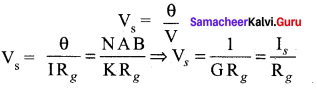Question 4. Define in terms of Current Sensitivity of a galvanometer.
Answer: It is defined as the deflection produced per unit current flowing through it. Is = θI = NABK ⇒ Is = 1G

Magnetism and Magnetic Effects of Electric Current 5-Marks Questions

Question 1. Derive an expression for potential energy of a bar magnet in a uniform magnetic field.
Answer: When a bar magnet (magnetic dipole) of dipole moment B⃗ m is held at an angle 0 with the direction of a uniform magnetic field B , the magnitude of the torque acting on the dipole is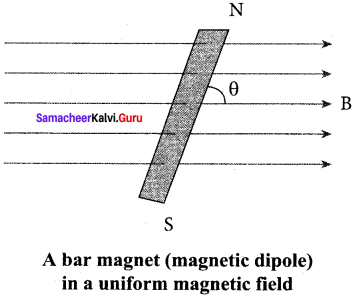|τ⃗ B| = ∣∣P⃗ B∣∣ ∣∣B⃗ ∣∣ sin θ ….. (1) If the dipole is rotated through a very small angular displacement dθ against the torque τB at constant angular velocity, then the work done by external torque (τ⃗ ext) for this small angular displacement is given by dW = (τ⃗ ext) dθ …… (2) The bar magnet has to be moved at constant angular velocity, which implies that |τ⃗ B| = |τ⃗ ext| dW = Pm B sin θ d θ Total work done in rotating the dipole from θ’ to θ is e e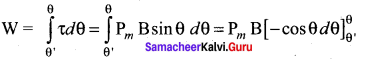W = -Pm B (cosθ – cosθ’) …… (3) This work done is stored as potential energy in bar magnet at an angle θ when it is rotated from θ’ to θ and it can be written as U = -Pm B (cosθ – cosθ’) …(4) In fact, the equation (4) gives the difference in potential energy between the angular positions θ’ and θ. We can choose the reference point θ’ = 90°, so that second term in the equation becomes zero and the equation 4 can be written as U = -Pm B(cosθ) …(5) U = -Pm • B⃗  Case 1: (i) If θ = 0°, then U= —Pm B (cos 0° ) = Pm B (ii) If θ = 180°, then U=-pm B(cos 180°) = pm B The potential energy stored in a bar magnet in a uniform magnetic field is given by

Question 2. Write down the application of Hysteresis loop.
Answer: Applications of hysteresis loop: The significance of hysteresis loop is that it provides information such as retentivity, coercivity, permeability, susceptibility and energy loss during one cycle of magnetisation for each ferromagnetic material. Therefore, the study of hysteresis loop will help us in selecting proper and suitable material for a given purpose. Some examples:

(i) Permanent magnets: The materials with high retentivity, high coercivity and high permeability are suitable for making permanent magnets. Examples: Steel and Alnico

(ii) Electromagnets: The materials with high initial permeability, low retentivity, low coercivity and thin hysteresis loop with smaller area are preferred to make electromagnets. Examples: Soft iron and Mumetal (Nickel Iron alloy).

(iii) Core of the transformer: The materials with high initial permeability, large magnetic induction and thin hysteresis loop with smaller area are needed to design transformer cores. Examples: Soft iron

Question 3. Write down the difference between soft and hard ferromagnetic materials.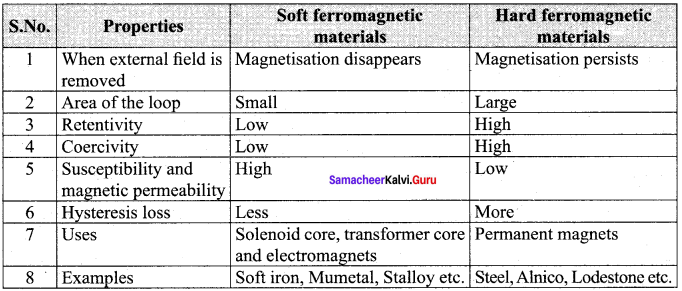Question 4. State and explain the Biot – Savart Law.
Answer: Biot and Savart experimentally observed that the magnitude of magnetic field dB⃗  at a point P at a distance r from the small elemental length taken on a conductor carrying current varies (i) directly as the strength of the current I (ii) directly as the magnitude of the length element dl→ (iii) directly as the sine of the angle (say,θ) between dl→ and r^. (iv) inversely as the square of the distance between the point P and length element dl→. This is expressed as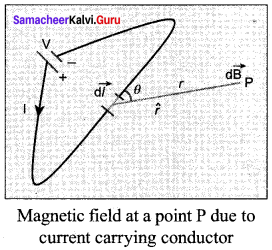dB ∝ Idlr2 sin θ dB = k Idlr2 sin θ Where K = μ04π in SI units and k = 1 in CGS units. In vector notation, dB⃗  = μ04π Idl⃗ ×r^r2 ….. (1) Here vector dB⃗  is perpendicular to both I dl→ (pointing current carrying conductor the direction of current flow) and the unit vector and r^ directed from dl→ toward point P The equation 1 is used to compute the magnetic field only due to a small elemental length dl→ of the conductor. The net magnetic field at P due to the conductor is obtained from principle of superposition by considering the contribution from all current elements I dl→. Hence integrating equation (1), we get B⃗  = ∫ dB⃗  = µ0I4π ∫ dl→×r^r2 ……. (2) where the integral is taken over the entire current distribution. Case: 1. If the pont P lies on the ckonductor, then θ = 0°. Therefore, dB⃗  is zero. 2. If the point lies perpendicular to the conductor, then θ = 90°. Therefore, dB⃗  is maximum and is given by dB⃗  = Idlr2 n^. where n^ is the unit vector perpendicular to both I dl→ and $$\hat{r$$.

Question 5. Write down the difference between Coulomb’s Law and Biot-Savart Law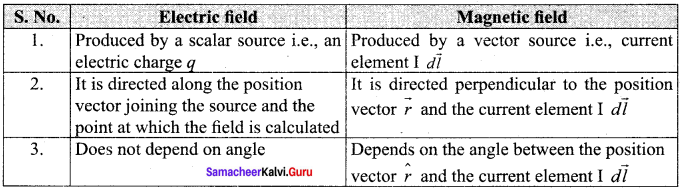Question 6. Obtain an expression for the magnetic dipole moment of a revolving electron.
Answer: Magnetic dipole moment of revolving electron: Suppose an electron undergoes circular motion around the nucleus. The circulating electron in a loop is like current in a circular loop (since flow of charge is current). The magnetic dipole moment due to current carrying circular loop is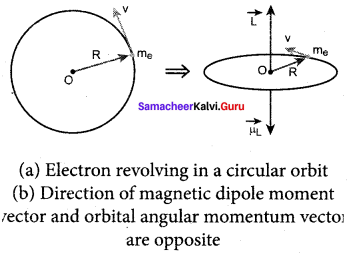µ⃗ L = A→ ….. (1) In magnitude, µ = I A If T is the time period of an electron, the current due to circular motion of th electron is I = −eT ……. (2) where -e is the charge of an electron. If R is the radius of the circular orbit and v is the velocity of the electron in the circular orbit, then T = 2πRv …… (3) Using equation (2) and equation (3) in equation (1), we get µL = –e2πRv πR2 = evR2 ……. (4) Where A = πR2 is the area of the circular loop. By definetion, angular moment of the electron about O is L→ = R→ x P→ In magnitude, L = PR = mvR …….. (5) Using equation (4) and equation (5), we get μLL = –evR2mvR = e2m L→ ……. (6) The negative sign indicates that the magnetic moment and angular momentum are in opposite direction. In magnitude, μLL = e2m = 1.60×10−192×9.11×10−31 = 0. 0878 x 1012 μLL = 8.78 x 1010 C kg-1 = content The ration μLL is a constant and also known as gyro-magnetic ration μLL. It must be noted that the gyro-magnetic ratio is a constant of proportionality which connects angular momentum of the electron and the magnetic moment of the electron. According to Neil’s Bohr quantization rule, the angular momentum of an electron moving in a stationary orbit is quantized, which means, l = nh = n h2π where, h is the planck’s constant (h = 6.63 x 10-34 J s) and number n takes natural numbers (i.e., n = 1,2,3,…..). Hence,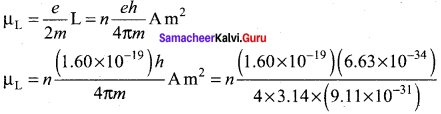µL = n x 9.27 x 10-24 A m2 The minimum magnetic moment can be obtained by subsituting by sustituting n = 1, µL = n x 9.27 x 10-24 A m2 = 9.27 x 10-24 J T-1 = (µL)min = µB Where, µB = eh4πm = 9.27 x 10-24 A m2 is called Bohr magneton. This is a convenient unit with which one can measure atomic magnetic moments.

Question 7. Apply Ampere’s Circuital Law to find the magnetic field both inside and outside of a toroidal solenoid.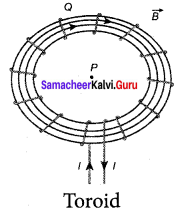Answer: A solenoid is bent in such a way its ends are joined together to form a closed ring shape, is called a toroid. The magnetic field has constant magnitude inside the toroid whereas in the interior region (say, at point P) and exterior region (say, at point Q), the magnetic field is zero.

(a) Open space interior to the toroid: Let us calculate the magnetic field Bp at point P. We construct an Amperian loop 1 of radius r1 around the point P. For simplicity, we take circular loop so that the length of the loop is its circumference.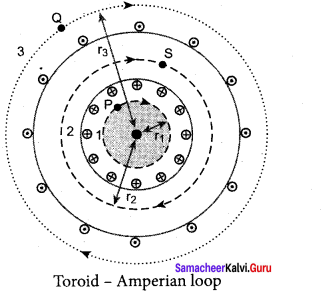L1 = 2π1 Ampere’s circuital law for the loop 1 is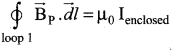Scince, the loop 1 encloses no current, Ienclosed = 0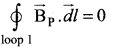This is possible only if the magnetic field at point P vanishes i.e. B⃗ p = 0

(b) Open space exterior to the toroid: Let us calculate the magnetic field BQ at point Q. We construct an Amperian loop 3 of radius r3 around the point Q. The length of the loop is L3= 2πr3 Ampere’s circuital law for the loop 3 is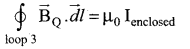Since, in each turn of the toroid loop, current coming out of the plane of paper is cancelled by the current going into the plane of paper. Thus, Ienclosed = 0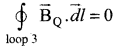This is possible only if the magnetic field at point Q vanishes i.e. B⃗ Q = 0

(c) Inside the toroid: Let us calculate the magnetic field BS at point S by constructing an Amperian loop 2 of radius r2 around the point S. The length of the loop is L2= 2πr2 Ampere’s circuital law for the loop 2 is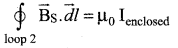Let I be the current passing through the toroid and N be the number of turns of the toroid, then Ienclosed = NI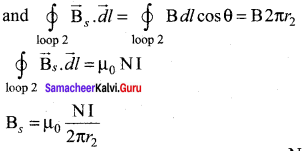The number of turns per unit length is n = n2πr2 then the magnetic field at point S is BS = μ0nI

Question 8. Obtain an expression for force on a moving charge in a magnetic field.
Answer: When an electric charge q is moving with velocity v⃗  in the magnetic field B⃗ , it experiences a force, called magnetic force F⃗ m. After careful experiments, Lorentz deduced the force experienced by a moving charge in the magnetic field F⃗ m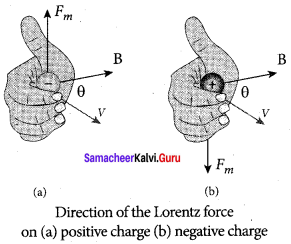F⃗ m = q(v⃗  x B⃗ ) ……. (1) In magnitude, Fm=qvB sin θ ……. (2) The equations (1) and (2) imply

1. F⃗ m is directly proportional to the magnetic field B⃗
2. F⃗ m is directly proportional to the velocity v⃗
3. F⃗ m is directly proportional to sine of the angle between the velocity and magnetic field
4. F⃗ m is directly proportional to the magnitude of the charge q
5. The direction of F⃗ m is always perpendicular to v⃗  and g as F⃗ m is ti’e cross product of v⃗  and B⃗
6. The direction of jprn is on negative charge is opposite to the direction of F charge provided other factors are identical.
7. If velocity v of the charge q is along magnetic field B⃗  then, F⃗ m is zero.

Magnetism and Magnetic Effects of Electric Current Numerical Problems

Question 1. The radius of the first orbit of hydrogen atom is 0.5 Å. The electron moves in an orbit with a uniform speed of 2.2 x 106 ms-1. What is the magnetic field produced at the centre of the nucleus due to the motion of this electron? Use µ0 = 4π x 10-7 Hm-1 and electric charge = 1.6 x 10-19C Solution: Here, r = 0.5 Å = 0.5 x 10-10m v = 2.2 x 106 ms-1 Period of revolution of electron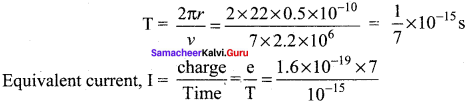= 1.12 x 10-3 A Magnetic field produced at the centre of the nucleus,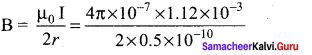B = 14. 07 T

Question 2. A positive charge of 1.5 µC is moving with a speed of 2 x 10-6 ms-1 along the positive X-axis. A magnetic field, B→ = (o.2 j^ + 0.4 k^) tesla acts in space. Find the magnetic force acting on the charge. Solution: Here q = 1.5 µC = 1.5 x 10-6C v⃗  = 2 x 10-6 i^ ms-1 ; B→ = (o.2 j^ + 0.4 k^) T Magnetic force on the positive charge is F⃗  = (v⃗  x B→) = 1.5 x 10-6 [2 x 106i^ x (o.2 j^ + 0.4 k^)] 3.0 [o.2 j^ x j^ + 0.4 i^ x k^] = (0.6 k^ – 1.2 j^) N [∴ i x j^ = k^, i^ x k^ = –j^]

Question 3. Copper has 8.0 x 1028 electrons per cubic metre carrying a current and lying at right angle to a magnetic field of strength 5 x 10-3 T. experiences a force of 8.0 x 10-2 N. Calculate the drift velocity of free electrons in the wire. Solution: n = 8 x 1028 m-3 ; l = lm A= 8 x 1028 m-2 ; e = 1.6 x 10-9C Total charge contained in the wire q = volume of wire x ne = Alne = 8 x 10-6 x 1 x 8 x1028 x 1.6 x 10-19C = 102.4 x 103 C If vd is the drift speed of electrons, then F = qvd B sin 90° = qvd B ∴ vd = FqB = 8.0×10−2102.4×103×5×10−3 ms-1 vd = 1.56 x 10-4 ms-1

Question 4. An electron is moving at 106 ms-1 in a direction parallel to a current of 5A flowing through an infinitely long straight wire, separated by a perpendicular distance of 10cm in air. Calculate the magnitude of the force experienced by the electron. Solution: Magnetic field of the straight wire carrying a current of 2 A, at a distance of 10cm or 0. lm from it is B = µ0I2πr = 4π×10−7×52π×0.1 = 10-5T This field acts perpendicular to the direction of the electron. So magnetic force on the electron is F = qv B sin 90° = 1.6 x 10-19 x 106 10-5 x 1 F = 1.6 x 10-18 N

Common Errors and Its Rectifications

Common Errors:

1. Students may not know about arrow mark in the figures, (diagram)
2. Students wrongly mention the unit of magnetic induction. Eg. 0.5 x 10-5 Tesla. Most of the children makes the mistakes in this area.
3. Student may confuse the direction of current versus produced magnetic induction.

Rectifications:

1. Arrow mark is importance to show where the electric and magnetic fields are directed.
2. The correct unit of magnetic induction of T (or) tesla.
3. If the current moves in upward the magnetic induction is anti-clock. If the current in downward, the magnetic induction is clockwise.

## How to Prepare using Samacheer Kalvi 12th Physics Chapter 3 Magnetism and Magnetic Effects of Electric Current Notes PDF?

Students must prepare for the upcoming exams from Samacheer Kalvi 12th Physics Chapter 3 Magnetism and Magnetic Effects of Electric Current Notes PDF by following certain essential steps which are provided below.

• Use Samacheer Kalvi 12th Physics Chapter 3 Magnetism and Magnetic Effects of Electric Current notes by paying attention to facts and ideas.
• Pay attention to the important topics
• Refer TN Board books as well as the books recommended.
• Correctly follow the notes to reduce the number of questions being answered in the exam incorrectly
• Highlight and explain the concepts in details.

## Frequently Asked Questions on Samacheer Kalvi 12th Physics Chapter 3 Magnetism and Magnetic Effects of Electric Current Notes

#### How to use Samacheer Kalvi 12th Physics Chapter 3 Magnetism and Magnetic Effects of Electric Current Notes for preparation??

Read TN Board thoroughly, make separate notes for points you forget, formulae, reactions, diagrams. Highlight important points in the book itself and make use of the space provided in the margin to jot down other important points on the same topic from different sources.

#### How to make notes for Samacheer Kalvi 12th Physics Chapter 3 Magnetism and Magnetic Effects of Electric Current exam?

Read from hand-made notes prepared after understanding concepts, refrain from replicating from the textbook. Use highlighters for important points. Revise from these notes regularly and formulate your own tricks, shortcuts and mnemonics, mappings etc.
Share: